Hypothesis Testing in Normal Admixture Models to Detect Heterogeneous Genetic Signals | OMICS International
Journal of Biometrics & Biostatistics

Like us on:

# Hypothesis Testing in Normal Admixture Models to Detect Heterogeneous Genetic Signals

Qian Fan1, Richard Charnigo1*, Zohreh Talebizadeh2 and Hongying Dai3,4

1Department of Statistics, 725 Rose Street, University of Kentucky, Lexington KY, USA

2Division of Experimental and Translational Genetics, 2401 Gillham Road, Children’s Mercy Hospital, Kansas City MO, USA

3Research Development and Clinical Investigation, 2401 Gillham Road, Children’s Mercy Hospital, Kansas City MO, USA

4Department of Biomedical & Health Informatics, 5100 RockHill Road, University of Missouri-Kansas City, Kansas City MO, USA

*Corresponding Author:
Richard Charnigo
Department of Statistics
725 Rose Street, University of Kentucky
Lexington KY, USA
Tel: +859-218-2072
E-mail: [email protected]

Received date: July 31, 2014; Accepted date: October 08, 2014; Published date: October 15, 2014

Citation: Fan Q, Charnigo R, Talebizadeh Z, Dai H (2014) Hypothesis Testing in Normal Admixture Models to Detect Heterogeneous Genetic Signals. J Biom Biostat S12:005. doi:10.4172/2155-6180.S12-005

Copyright: © 2014 Fan Q, et al. This is an open-access article distributed under the terms of the Creative Commons Attribution License, which permits unrestricted use, distribution, and reproduction in any medium, provided the original author and source are are credited.

Visit for more related articles at Journal of Biometrics & Biostatistics

#### Abstract

In this work we consider a three-component normal mixture model in which one component is known to have mean zero and the other two contaminating components have a nonnegative and a no positive mean respectively, while all three components share a common unknown variance parameter. One potential application of this model may be in prioritizing statistical scores obtained in biological experiments, including genetics data. Such a mixture model may be useful in describing the distribution of numerous Z test statistics corresponding to different genes or SNPs, such that a “significant” Z test statistic for a particular gene suggests its connection to a medical condition. More specifically, the inferences drawn from such a mixture model may be useful in a filtration algorithm to remove large subsets of genes or SNPs from consideration, thereby reducing the need for stringent and power-depleting multiplicity adjustments for controlling type I error rates on the remaining genes. We show how to test whether there is contamination in at least one direction (i.e., the mixture model truly requires at least two components) and, if so, how to test whether there is contamination in both directions (i.e., the mixture model truly requires all three components). We assess our testing procedures in simulation studies and illustrate them through application to LOD scores in a genome-wide linkage analysis from an autism study.

#### Keywords

Autism; Bilaterally contaminated normal model; Gene expression; Genome-wide linkage; LOD; Microarray; Nuisance parameter; Omnibus null hypothesis; Unilateral null hypothesis

#### Introduction

Suppose that X1, X2. . . Xn are independent and identically distributed (iid) with probability density function.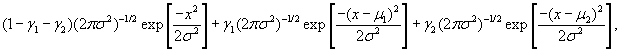(1)

a three-component normal mixture model in which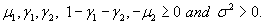If σ2 is known while the other parameters are unknown, then (1) is called a bilaterally contaminated normal (BCN) model. Charnigo et al.  observed that, in this case, one may test the omnibus null hypothesis that γ1μ1 = 0 and γ2μ2=0 by comparing the second sample moment to a known multiple of a chi-square quantile. This omnibus null hypothesis states that the BCN model reduces to a standard normal distribution.

Moreover, if the omnibus null hypothesis is false, then one may test the unilateral null hypothesis that γ1μ1 = 0 or γ2μ2=0by defining a Wald-type test statistic based on a quadratic function of σ2 and the first three moments. This unilateral null hypothesis states that there is contamination by either a positive-mean normal distribution or a negative-mean normal distribution but not both simultaneously.

If σ2 is also unknown, then (1) is called a bilaterally contaminated normal with nuisance parameter (BCN+NP) model. This case was noted but not studied by Charnigo et al.  or to our knowledge any other authors, and poses greater challenges to testing the omnibus null hypothesis and unilateral null hypothesis. The omnibus null hypothesis for either the BCN model or the BCN+NP model suffers from a nonidentify ability of parameters that is characteristic of mixture modeling. For instance, there are infinitely many possibilities for γ1 when μ1 is 0, and vice versa. In fact, even the unilateral null hypothesis has an ambiguous parametric representation. Thus, the assumptions justifying the standard chi-square theory for likelihood ratio testing are not met for either the BCN or BCN+NP model [2-5].

Due to difficulties with likelihood ratio testing in mixture modeling, Chen et al.  developed a modified likelihood ratio test to address whether a two-component mixture could be reduced to a homogeneous distribution. Applicable under fairly general circumstances, their test was supported by an asymptotic theory and simulation results showing that chi-square quantiles could be used as critical values. Dai and Charnigo [7,8] subsequently adapted the modified likelihood ratio test to accommodate two-component mixtures in which some or all of the parameters for one mixture component were known a priori. They referred to such a mixture model as a contaminated density model or, for short, contaminated model. Modified likelihood ratio testing for mixture models with more than two components does not appear to have a similarly tractable asymptotic theory, which has inspired the development of the EM-test [9-11]. While we have some optimism that the EM-test may be useful for inference in the BCN+NP model, the present paper will develop tests based primarily on moments.

The practical motivation for the BCN+NP model is largely the same as for the BCN model, as described by Charnigo et al. . To briefly recap, suppose that Xi is a test statistic for comparing cases to controls on the expression level of gene i in a microarray [12,13], such that Xi ~ N(0, σ2) if patients and controls have the same mean

expression level, Xi ~ N(μ12) if patients have greater expression, and Xi ~ N(μ22) if patients have lesser expression. Testing the omnibus null hypothesis asks whether some genes are differentially expressed in patients versus controls, and testing the unilateral null hypothesis asks whether there exist simultaneously some genes that are over expressed and other genes that are under expressed.

As suggested by Dai and Charnigo  in a somewhat different context, omnibus testing may help a researcher to avoid overly stringent adjustments for controlling Type I error rates in multiple testing. For example, if an omnibus test suggests no differential expression within a subset of genes, then that subset of genes can be “filtered out” and not contribute to the adjustments for controlling Type I error rates in other gene-specific tests across the genome. Thus, gene-specific tests in subsets of genes that have not been filtered out may become more powerful, improving the researcher’s ability to detect differential expression within such subsets of genes. As an aside, we mention that gene expression data are ordinarily normalized prior to performing any test comparing cases to controls. Evaluating or developing methodology for normalization is, however, beyond the scope of the present paper. Our real data example in this paper will pertain to LOD scores rather than to gene expression data; thus, normalization is not applicable

Assuming that σ2 is known to equal 1 may seem reasonable in some contexts. However, the BCN model may fit some real-world data sets rather poorly, in which case one would have lesser confidence in inferences based on the BCN model. The BCN+NP model may still represent an imperfect approximation to reality, but the nuisance parameter σ2 may absorb some lack-of-fit from the BCN model, so that one could have greater confidence in inferences based on the BCN+NP model.

The rest of this paper is organized as follows. Section 4 presents our procedure for testing the omnibus null hypothesis in the BCN+NP model, which is based on a union-intersection test that examines both odd sample moments and ratios of even sample moments. Section 5 describes our method for testing the unilateral null hypothesis, which employs an auxiliary estimator of σ2and uses an upper bound for the error in that estimator to adjust the critical value for a Wald-type statistic. Simulation results appear in Section 6, portraying the actual Type I and Type II error rates of both tests under their respective null and alternative hypotheses. Section 7 features a case study, in which the BCN+NP model is compared to the BCN model on real genomewide linkage data. Our conclusions, including a discussion of future research, appear in Section 8. The R code to implement our testing procedures is available upon request to the corresponding author.

#### Testing the Omnibus Null Hypothesis

In this section we are concerned with testing the omnibus null hypothesis against its corresponding alternative,

H0: γ1μ1=0 and γ2μ2=0 against H1: γ1μ1≠0 or γ2μ2≠0.

The alternative hypothesis indicates that there is contamination in at least one direction.

If σ2 were known, a simple test would be obtained by comparing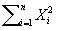to σ2 times an upper quantile of a chi-square distribution . However, since σ2is unknown, a test statistic involvingmust be normalized to address the dependence of its distribution on σ2.

For example, the test statistic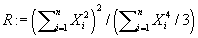has a distribution that does not depend on σ2. In fact, the asymptotic null distribution of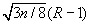is standard normal by Cramer’s Theorem . This seems to suggest that we reject the null hypothesis when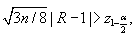where α is the desired significance level. Unfortunately, such a procedure is not consistent against all alternative hypotheses. Indeed, R may converge in probability to a numberless than 1 under some alternative hypotheses (e.g., when σ2=1, μ1=1, μ2=−2, γ12=0.05), to1 under others (e.g., when σ2=1,μ1=2,μ2=−2, γ12=1/6), or even to a number greater than1 (e.g., when σ2=1, μ1=1, μ2=−1, γ12=0.3).

The above example does not prove the non-existence of a simple test based on moments, but other obvious candidates for a test statistic involving low-order moments suffer similar difficulties. On the other hand, as Lemma 4.1 shows, H0 can be equivalently expressed as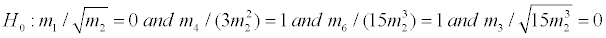where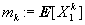is the kth moment of X1.

Lemma 4.1 If H0 is false, then at least one of the following conditions must hold(a)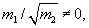(b)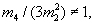(c)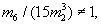(d)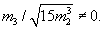Proof. First suppose that H0 is false with μ1= −μ2> 0 and γ1= γ2> 0. If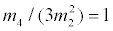, then γ1=1/6 and thusNext suppose that H0 is false with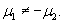If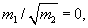then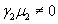and thus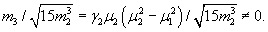This motivates the development of a union-intersection test . Let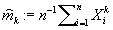denote the kth sample moment and put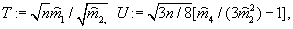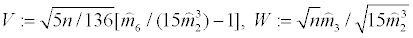Theorem 4.1 establishes a test based on T, U, V, and W.

Theorem 4.1 Let α1, α2, α3, α4, α ∈(0, 1) be given such that α=α1234. Consider a test that rejects H0 if and only if at least one of the following conditions holds:(a)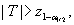(b)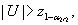(c)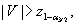(d)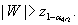. Then the probability of incorrectly rejecting the null hypothesis under H0 is asymptotically less than or equal to α, while the probability of correctly rejecting the null hypothesis under H1 converges to 1.

Proof. First suppose that H0 is true. In this case, T, U, V, and W are all asymptotically standard normal by Cramer’s Theorem. The probability of incorrectly rejecting the null hypothesis is bounded above by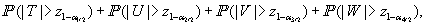which converges to α1234=α.

Next suppose that H0 is false. In this case, at least one of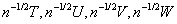converges in probability to a nonzero quantity. Thus, at least one of |T|, |U|, |V|, and |W| diverges to infinity in probability, so that the corresponding probability in the preceding paragraph tends to 1, and this probability is a lower bound for the probability of correctly rejecting the null hypothesis.

We note that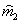in the definitions of T and W may be replaced by the familiar sample variance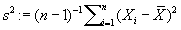without disturbing the conclusions of Theorem 1.

A question of practical interest is how to choose α1, α2, α3, α4,. A simple choice is to set each of them equal to α/4, but this may not optimize the power to correctly reject H0. For example, if there is bilateral contamination and the contamination is symmetric, then neither T nor W will be useful. Rather, the contamination will be detectable via U or V, suggesting that α2 and α3 be chosen close to α/2 while α1 and α4 be taken negligibly small.

Thus, if a data analyst has reason to anticipate a certain type of contamination, then he/she may choose α1, α2, α3, α4,accordingly. The choice of α1, α2, α3, α4,is explored empirically in the simulation studies of Section 6. However, we also caution against choosing α1, α2, α3, α4, after examining the data, as this may result in a hidden inflation of Type I error rate.

#### Testing the Unilateral Null Hypothesis

Now we are concerned with testing the unilateral null hypothesis against its corresponding alternative,

H0: γ1μ1=0 or γ2μ2=0 against H1: γ1μ1≠0 and γ2μ2≠0.

The alternative hypothesis indicates that there is contamination in both directions. Because this testis developed assuming that the omnibus null hypothesis is false, in practice we recommend sequential testing: first perform the test described in Section 4, and then, only if the omnibus null hypothesis is rejected, proceed to the test that we describe presently.

If σ2 were known, a test of the unilateral null hypothesis could be obtained  based on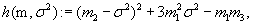Where m: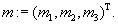. More specifically, since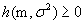with equality if and only if H0 is true, one could reject H0 if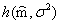exceeded a critical value suggested by Cramer’s Theorem, where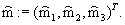However, since σ 2 is unknowncannot be calculated and must be replaced by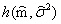for some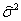.The question then becomes, what is an appropriate critical value ?

Before addressing the question, some comment on the choice ofis warranted. In the simulation studies of Section 6, we taketo be the maximum likelihood estimator of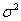. If H1 is indeed true (and a compact parameter space is imposed), thenis anticipated to converge toat the rate of n−1/2. If H0 is true, thenis anticipated to converge at the slower rate of n−1/4 . However, maximum likelihood estimation ofis not essential. Rather, we assume thatis chosen such that there exists known δn with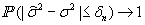and δn→ 0. For instance, ifconverges at a rate of n−1/4 or better, one may take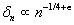for some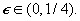The following lemmas are preparatory to identifying a critical value for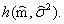In what follows, we define A to be the 3×3 matrix with Aij=mij−mimj and b to be the vector of partial derivatives of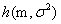with respect to m. We also define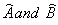to be the corresponding estimators.

Lemma 5.1 Suppose H0 is true. Then, for any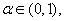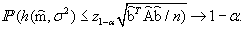Proof. By Cramer’s Theorem,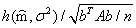converges in law to standard normal. Since A and b are continuous functions of m and, and since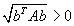when the omnibus null hypothesis is false, the Continuous Mapping Theorem implies that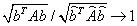in probability. By Slutsky’s Theorem,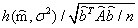converges in law to standard normal. The desired result is an immediate consequence.

Lemma 5.2 Suppose H0is true. Then,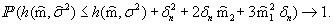Proof. Suppose that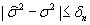and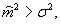, which occur with probability approaching 1.

Then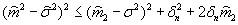and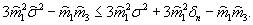Adding these two inequalities yields the desired result.

We are now in a position to describe our testing procedure.

Theorem 5.1 Let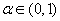be given. Consider a test that rejects H0 if and only if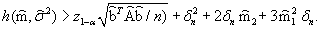Then the probability of incorrectly rejecting the null hypothesis under H0 is asymptotically less than or equal to α, while the probability of correctly rejecting the null hypothesis under H1 converges to 1.

Proof. The first part of the conclusion follows from Lemma 5.1 and Lemma 5.2. The second part of the conclusion follows from the facts thatconverges in probability to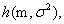the latter quantity is positive under H1 , and the critical value converges in probability to 0.

Our guidelines for δn are asymptotic. However, to carry out the test in practice, one must specify δn for a finite sample. If δn is too small, then the Type I error rate of the test will be too large. If δn is too large, then the power of the test will be unnecessarily low. The choice of δn is explored empirically in the simulation studies of Section 6.

#### Simulation Results

First we present results from simulation studies to assess the Type I and Type II error rates of our omnibus testing procedure in finite samples from the BCN+NP model. Table 1 pertains to omnibus testing based on sample sizes of n=100 and n=1000, with α1234=0.0125 and parameter values as shown in the left column. (We put σ2=1 to generate data but subsequently treated σ2 as unknown. The simulation size was 1000.) To illustrate use of Table 1, consider two examples: First, when n=100, there is approximately a 3.8% Type I error rate. Second, when n=1000, there is approximately 78.0% power against the specific alternative (μ1, μ2, γ12)=(0,-1, 0.2, 0.1).

1, µ2, γ1, γ2 ) n=100 n=1000
(0,0,0,0) 0.038 0.038
(1, -1, 0.1, 0.1)
(1, -1, 0.2, 0.2)
(2, -2, 0.1, 0.1)
(2, -2, 0.2, 0.2)
0.050
0.021
0.092
0.015
0.054
0.033
0.521
0.046
(1, 0, 0.2, 0.1)
( 0, -1, 0.2, 0.1)
(2, 0, 0.2, 0.1)
( 0, -2, 0.2, 0.1)
0.291
0.091
0.845
0.408
1.000
0.780
1.000
1.000
(1, -1, 0.2, 0.1)
(1, -2, 0.1, 0.1)
(2, -2, 0.2, 0.1)
(2, -1, 0.2, 0.2)
0.096
0.170
0.137
0.256
0.657
0.979
0.975
1.000

Table 1: Type I Error and Power for BCN+NP Data: α1234=0.0125.

Tables 2 and 3 also pertain to omnibus testing but with different choices of α1to α4. In Table 2 more consideration for rejecting the omnibus null hypothesis is given to T and W, whereas in Table 3 more consideration is given to U and V.

1, µ2, γ1, γ2 ) n=100 n=1000
(0,0,0,0) 0.037 0.046
(1, -1, 0.1, 0.1)
(1, -1, 0.2, 0.2)
(2, -2, 0.1, 0.1)
(2, -2, 0.2, 0.2)
0.038
0.037
0.079
0.029
0.054
0.042
0.384
0.039
(1, 0, 0.2, 0.1)
( 0, -1, 0.2, 0.1)
(2, 0, 0.2, 0.1)
( 0, -2, 0.2, 0.1)
0.353
0.101
0.902
0.443
1.000
0.854
1.000
1.000
(1, -1, 0.2, 0.1)
(1, -2, 0.1, 0.1)
(2, -2, 0.2, 0.1)
(2, -1, 0.2, 0.2)
0.102
0.182
0.191
0.275
0.720
0.988
0.978
1.000

Table 2: Type I Error and Power for BCN+NP Data: α14=0.02, α23=0.005.

1, µ2, γ1, γ2 ) n=100 n=1000
(0,0,0,0) 0.032 0.038
(1, -1, 0.1, 0.1)
(1, -1, 0.2, 0.2)
(2, -2, 0.1, 0.1)
(2, -2, 0.2, 0.2)
0.033
0.024
0.080
0.002
0.056
0.024
0.507
0.055
(1, 0, 0.2, 0.1)
( 0, -1, 0.2, 0.1)
(2, 0, 0.2, 0.1)
( 0, -2, 0.2, 0.1)
0.197
0.073
0.730
0.319
1.000
0.661
1.000
1.000
(1, -1, 0.2, 0.1)
(1, -2, 0.1, 0.1)
(2, -2, 0.2, 0.1)
(2, -1, 0.2, 0.2)
0.045
0.138
0.088
0.135
0.541
0.958
0.946
0.999

Table 3: Daytime high temperature 3-day forecast vs actual, ranked by the
standard deviations of forecast errors.

As shown in Tables 1-3, the omnibus test appears conservative at both sample sizes and all three combinations of α1 through α4, in that the observed Type I error rate is less than 5%. As anticipated, power tends to be greater with a larger sample size. The power under unilateral contamination is better when the contaminating mean is larger (± 2) rather than smaller (± 1) and when the weight of contamination is larger (0.2) rather than smaller (0.1). The power under asymmetric bilateral normal contamination tends to be better when the contaminating means are different (in absolute value) than when the weights are different. The power under symmetric bilateral contamination is relatively low except when the sample size is larger and (μ1, μ2, γ12)=(2,−2, 0.1, 0.1).

For added realism, we also consider scenarios in which the BCN+NP model is mispecified, in that the data originate from the “bilaterally contaminated and scaled T model with nuisance parameter”(BCT+NP model) but then are subjected to forward and reverse cumulative distribution function(cdf) transformations.

More specifically, suppose that T1,. . ., Tn are i id with pdf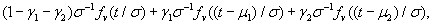Where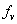denotes the T pdf on ν degrees of freedom anddenotes the corresponding cdf. We assume that ν is known; in the context of microarray data analysis, ν will relate to the numbers of persons (or experimental units) on which gene expression data have been obtained. Data arising from this model can be transformed by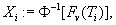where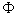is the standard normal cdf. The transformed data are then analyzed as if they had arisen from the BCN+NP model. If σ2=1 and γ1μ12μ2=0, then the transformed data will actually be standard normal (and hence from the BCN+NP model), but otherwise the transformed data may not truly be from the BCN+NP model.

Table 4 is organized analogously to Table 1, except that there are additional columns corresponding to various choices of ν from 5 to 100. (We have also obtained results analogous to those in Tables 2 and 3 but have omitted including them in tabular form to streamline this manuscript.) The omnibus test appears conservative in most scenarios and is not markedly anticonservative in any. As anticipated, power tends to be greater with a larger sample size. The power under unilateral contamination tends to be greater if either the contaminating mean or the contaminating weight is larger, and the power seems to increase with the degrees of freedom. The power under asymmetric bilateral contamination often increases with the degrees of freedom and tends to be smaller when both contaminating means are smaller; the power is very sensitive to the degrees of freedom when both contaminating weights are smaller. The choice (α1, α2, α3, α4)=(0.02, 0.005, 0.005, 0.02) seems best overall. The power under symmetric bilateral contamination actually decreases with the degrees of freedom in many scenarios and tends to be smaller when both contaminating means are smaller. The choice (α1, α2, α3, α4)=(0.005, 0.02, 0.02, 0.005) appears best overall.

1, µ2, γ1, γ2 ) n=100 n=1000
df=5 10 50 100 df=5 10 50 100
(0,0,0,0) 0.027 0.03 0.026 0.039 0.038 0.037 0.045 0.051
(1, -1, 0.1, 0.1)
(1, -1, 0.2, 0.2)
(2, -2, 0.1, 0.1)
(2, -2, 0.2, 0.2)
0.014
0.022
0.010
0.014
0.017 0.012 0.010 0.013 0.033 0.016 0.042 0.015 0.035 0.019 0.037 0.010 0.077 0.528 0.663 1.000 0.037 0.236 0.058 1.000 0.038 0.030 0.110 0.319 0.049 0.024 0.264 0.132
(1, 0, 0.2, 0.1)
(0, -1, 0.2, 0.1)
(2, 0, 0.2, 0.1)
(0, -2, 0.2, 0.1)
0.182
0.060
0.528
0.143
0.233 0.078 0.628 0.197 0.248 0.088 0.803 0.341 0.283 0.091 0.820 0.403 0.992 0.560 1.000 0.979 0.997 0.592 1.000 0.996 1.000 0.725 1.000 1.000 1.000 0.740 1.000 1.000
(1, -1, 0.2, 0.1)
(1, -2, 0.1, 0.1)
(2, -2, 0.2, 0.1)
(2, -1, 0.2, 0.2)
0.046
0.026
0.092
0.089
0.050 0.049 0.106 0.096 0.065 0.103 0.137 0.172 0.077 0.140 0.127 0.203 0.554 0.539 0.998 0.999 0.601 0.686 0.985 0.998 0.614 0.934 0.971 0.999 0.621 0.955 0.966 1.000

Table 4: Type I Error and Power for Transformed BCT+NP Data: α1234=0.0125.

Next we present results from simulation studies to assess the Type I and Type II error rates of our unilateral testing procedure in finite samples. Table 5 pertains to unilateral testing based on sample sizes of n=100 and n=1000 drawn from the BCN+NP model, with a “small” δ equaling 0.05 at the former sample size and 0.025 at the latter. (Note that (1000/100) −1/4 is close to 0.025/0.05.) Tables 6 and 7 double and triple the values of δ respectively.

1, µ2, γ1, γ2 ) n=100 n=1000
(0,0,0,0) 0.032 0.038
(1, -1, 0.1, 0.1)
(1, -1, 0.2, 0.2)
(2, -2, 0.1, 0.1)
(2, -2, 0.2, 0.2)
0.166
0.209
0.065
0.402
0.210
0.459
0.007
0.862
(1, 0, 0.2, 0.1)
( 0, -1, 0.2, 0.1)
(2, 0, 0.2, 0.1)
( 0, -2, 0.2, 0.1)
0.145
0.159
0.054
0.060
0.132
0.143
0.017
0.012
(1, -1, 0.2, 0.1)
(1, -2, 0.1, 0.1)
(2, -2, 0.2, 0.1)
(2, -1, 0.2, 0.2)
0.215
0.099
0.176
0.245
0.288
0.133
0.124
0.893

Table 5: Type I Error and Power for BCN+NP Data: Small δ.

1, µ2, γ1, γ2 ) n=100 n=1000
(1, -1, 0.1, 0.1)
(1, -1, 0.2, 0.2)
(2, -2, 0.1, 0.1)
(2, -2, 0.2, 0.2)
0.082
0.122
0.033
0.329
0.051
0.194
0.000
0.710
(1, 0, 0.2, 0.1)
( 0, -1, 0.2, 0.1)
(2, 0, 0.2, 0.1)
( 0, -2, 0.2, 0.1)
0.063
0.062
0.024
0.028
0.025
0.022
0.0030
.001
(1, -1, 0.2, 0.1)
(1, -2, 0.1, 0.1)
(2, -2, 0.2, 0.1)
(2, -1, 0.2, 0.2)
0.134
0.060
0.124
0.159
0.079
0.017
0.035
0.701

Table 6: Type I Error and Power for BCN+NP Data: Medium δ.

1, µ2, γ1, γ2 ) n=100 n=1000
(1, -1, 0.1, 0.1)
(1, -1, 0.2, 0.2)
(2, -2, 0.1, 0.1)
(2, -2, 0.2, 0.2)
0.032
0.068
0.026
0.279
0.007
0.050
0.000
0.545
(1, 0, 0.2, 0.1)
( 0, -1, 0.2, 0.1)
(2, 0, 0.2, 0.1)
( 0, -2, 0.2, 0.1)
0.010
0.023
0.014
0.007
0.007
0.002
0.001
0.000
(1, -1, 0.2, 0.1)
(1, -2, 0.1, 0.1)
(2, -2, 0.2, 0.1)
(2, -1, 0.2, 0.2)
0.054
0.031
0.100
0.096
0.016
0.001
0.010
0.428

Table 7: Type I Error and Power for BCN+NP Data: Large δ.

The middle four rows of Tables 5-7 indicate that Type I error rates are well controlled for medium to large δ and for small δ when the unilaterally contaminating mean is larger (±2) rather than smaller (±1). The other eight rows of Tables 5-7 reveal that power tends to be greater when both contaminating weights are larger (±0.2) rather than smaller (±0.1) and the sample size is larger. Of course, power is also a decreasing function of δ.

Table 8 is organized analogously to Table 5 but is based on transformed data from the BCT+NP model with degrees of freedom as indicated in column headings. (We have also obtained results analogous to those in Tables 6 and 7). Type I error rates are reasonably well controlled for medium to large δ when the degrees of freedom are larger. A larger δ than considered in these simulation studies would be necessary to control Type I error rates when the degrees of freedom are smaller, particularly when the unilaterally contaminating mean is larger (±2) rather than smaller (±1). Power is often larger when the degrees of freedom are smaller, but this is potentially misleading since the Type I error rates tend to be inflated as well. When the degrees of freedom are larger, the power tends to be greater when both contaminating weights are larger (±0.2) rather than smaller (±0.1) and the sample size is larger.

1, µ2, γ1, γ2 ) n=100 n=1000
df=5 10 50 100 df=5 10 50 100
(1, -1, 0.1, 0.1)
(1,-1, 0.2, 0.2)
(2, -2, 0.1, 0.1)
(2, -2, 0.2, 0.2)
0.312 0.508 0.596 0.951 0.261 0.428 0.357 0.875 0.190 0.278 0.100 0.552 0.193 0.270 0.088 0.455 0.829
0.994
0.999
1.000
0.662
0.977
0.867
1.000
0.338
0.648
0.027
0.996
0.266
0.591
0.020
0.975

(1, 0, 0.2, 0.1)
(0, -1, 0.2, 0.1) (2, 0, 0.2, 0.1)
(0, -2, 0.2, 0.1)

0.271 0.226 0.508 0.327 0.241 0.189 0.309 0.196 0.161 0.168 0.098 0.059 0.168 0.158 0.057 0.065 0.736
0.502
0.996
0.793
0.539
0.391
0.885
0.523
0.181
0.229
0.104
0.065
0.152
0.187
0.044
0.027
(1, -1, 0.2, 0.1) (1, -2, 0.1, 0.1) (2, -2, 0.2, 0.1) (2, -1, 0.2, 0.2) 0.417 0.443 0.822 0.807 0.346 0.290 0.644 0.677 0.243 0.140 0.261 0.365 0.220 0.113 0.183 0.313 0.968
0.980
1.000
1.000
0.873
0.885
1.000
1.000
0.444
0.309
0.518
0.985
0.351
0.198
0.294
0.941

Table 8: Type I Error and Power for Transformed BCT+NP Data: Small δ.

#### Case Study

To illustrate our new testing procedures, we analyzed LOD scores obtained in a whole genome linkage analysis from an autism study . Autism  is a complex neuro developmental condition that might be affected by multiple genetic and non-genetic factors. Furthermore, there is a high degree of phenotypic heterogeneity both within and among families. To address the heterogeneity in disease phenotypes, Talebizadeh et al.  proposed a novelmulti-step stratification method that divides subjects with autism into subgroups using previously developed cluster analyses of severity scores from an autism diagnostic test . The objective of the applied stratification method was to identify subgroups representing more homogeneous autism subjects by reducing both inter and intra-family heterogeneity. Linkage analysis  was then performed to identify genetic markers linked with autism within each subgroup. Linkage analysis is a method to find the approximate chromosomal position of disease genes by testing for co-segregation of a trait of interest relative to known genetic markers. The likelihood of co-segregation (linkage) is estimated by calculating LOD scores .

After data quality control and filtration, 16973 SNPs (autosomal and X-linked) from a total of 392 multiplex families were included for the linkage analysis. Subjects were stratified into a total of 16 subgroups considering the following: affected individual’s disease severity , intra-family heterogeneity, and affected individual’s gender [i.e., male only (M) and female-containing (Fc) pedigrees]. The LOD score from the linkage analysis is a measure of the strength of association between a genetic marker and disease in familial data. A LOD score that is less than or equal to 0 suggests no genetic linkage. To characterize the distribution of LOD scores, we applied the BCN+NP model to LOD scores within the “G4Fc” subgroup. The distributions of LOD scores in most other subgroups were not deemed suitable for BCN+NP modeling; they might have been amenable to a normal mixture model in which different components could have different variances, but such a model is beyond the scope of this manuscript.

The full BCN+NP model comprises three mixture components for LOD scores. The means of these mixture components are 0, μ1 ≥ 0, and μ2 ≤ 0. We assume that the within-component variance σ2 is unknown but equal across all components. We then applied our hypothesis testing procedures to detect whether there are substantial numbers of genetic variants from components witμ1 ≥ 0 orμ2 <0. The empirical distribution of the LOD values suggests either that there is some sort of digit preference in the data or that the underlying probability distribution is inherently of mixed type, with a discrete component supported on approximately 30 points and accounting for the vast majority of the observations. In either case, a histogram estimate suggests that approximating the empirical distribution of the LOD values using the BCN+NP model may be reasonable if one wishes the approximating distribution to be continuous (Cf. Figure 1).

To begin with, we note that σ2 in the BCN+NP model is estimated to be 0.119. Therefore, the BCN model with σ2 treated as known and equal to 1 would be highly inappropriate for these data and could yield faulty conclusions. In fact, since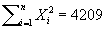is far less than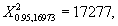naive omnibus testing for the BCN model using the procedure of Charnigo et al.  would fail to detect any heterogeneity.

On the other hand, omnibus testing for the BCN+NP model using the procedure introduced here in yields T=35.1, U=42.2, V=33.7, and W=5.5. Thus, the omnibus null hypothesis is decisively rejected for any reasonable choice of α, regardless of how α is divided among α1 through α4

Moving to unilateral testing for the BCN+NP model, we obtain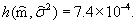With α=0.05 and δ=0, the critical value for rejection of the unilateral null hypothesis is 9.5×10−4. Since the critical value is an increasing function of δ (for example, the critical value with δ=0.05 is 3.1×10−3), there is no choice of δ for which the unilateral null hypothesis will be rejected at α=0.05.

To understand why the unilateral null hypothesis is not rejected, we can juxtapose the fitted BCN+NP model against the fitted “unilaterally contaminated normal model with nuisance parameter”(UCN+NP model), a special case of the BCN+NP model in which either γ1μ1=0 or γ2μ2=0 but not(necessarily) both. Both models are fitted by maximum likelihood and are displayed in Figure 1. They are numerically indistinguishable to three decimal places on the parameters, as 0.545N (0, 0.119) +0.135N (0.980, 0.119)+ 0.320N(0, 0.119) and 0.865N(0, 0.119)+ 0.135N(0.980, 0.119) respectively.

One might have anticipated that the estimate of μ2 equaling zero in the BCN+NP model should have forcedto equal zero as well. However, the former estimate is based on maximum likelihood, whereas the latter test statistic is based primarily on moments. Even so, neither the fitted BCN+NP model nor the test statistic argues against the unilateral null hypothesis.

In light of the simulation results in Section 6, one may also be concerned about the possibility of inadequate power for the unilateral testing procedure. However, because the sample size in this case study was more than 16 times the larger sample size from the simulation results, and because the estimate of μ2was zero, we do not believe that there was an undetected deviation of any importance from the unilateral null hypothesis in this case study, at least to the extent that the BCN+NP model approximation was valid.

The final fitted model for the G4Fc subgroup, one of the femalecontaining subgroups, is 0.865N (0, 0.119)+0.135N (0.980, 0.119). This suggests that about 13.5% of genetic variants belong to a mixture component with μ1>0. We further calculated the posterior probabilities for genetic variants belonging to this mixture component. Such a posterior probability is a monotone function of the LOD score but may provide some insight that a LOD score does not, namely the probabilistic interpretation of how likely the genetic variant is to belong to the mixture component with μ1>0.

There are 253 SNPs with posterior probability greater than 99% (corresponding LOD score, 1.27) and 669 SNPs with posterior probability above 98% (corresponding LOD score, 1.19). Using 50% posterior probability as a threshold, the cutoff point for LOD scores is 0.765. In other words, if a LOD score is less than 0.765, then the genetic variant will be assigned to the mixture component with mean zero. If a LOD score is greater than 0.765, then the genetic variant will be assigned to the mixture component with meanμ1>0. Alternatively, if one wishes to assign 13.5% of genetic variants to the mixture component with mean μ1>0, then one may use a cutoff of 0.427. On the other hand, a LOD score of 0.427, 0.765, or even 0.980 may not be sufficiently large to argue for a clear connection of the genetic variant with autism. Thus, caution is required in interpreting the results of the fitted model.

#### Conclusion

We have presented and theoretically justified new procedures for testing omnibus and unilateral null hypotheses in a bilaterally contaminated normal model with nuisance parameter representing the unknown within-component variability. As our case study makes clear, there will arise situations in which assuming the within-component variability to be known and equal to unity is not a viable modeling strategy, and thus the procedures in the earlier work by Charnigo et al.  will not be applicable.

Our case study also illustrates that having a unilateral testing procedure is worthwhile. One maybe inclined to assume that, if contamination is present in one direction, contamination should also be present in the other direction. Such an assumption may be true in many instances, but being able to declare that contamination is exclusively (or, at least, primarily) in one direction may be of scientific importance. Thus, even if one has an adequate sample size to estimate parameters for a model with two contaminating components, adopting such a model may be neither necessary nor desirable.

The primary limitation of the omnibus testing procedure proposed herein is that a union-inter section test with non-exclusive mechanisms to reject the null hypothesis (i.e., more than one of T through W could call for rejection simultaneously) will tend to be conservative. Even so, the simulation results suggest that the omnibus testing procedure may exhibit good power in many situations with unilateral contamination or asymmetric bilateral contamination. Symmetric bilateral contamination appears considerably more difficult to detect, presumably because such contamination is not easily distinguished from a larger value of the nuisance parameter under the omnibus null hypothesis. A secondary limitation is that the data analyst must specify α1 through α4. However, a “default” choice of α1234=α/4 may work reasonably well, if not optimally, in many situations.

The primary weakness of the unilateral testing procedure is its sensitivity to model misspecification. If the data originated from a bilaterally contained and scaled T model with nuisance parameter on low degrees of freedom and were transformed so that the bilaterally contaminated normal model with nuisance parameter could be applied, the Type I error rates may be surprisingly high. Of course, this can be corrected by adjusting δ, but we have not discovered a mechanism for adjusting δ under model misspecification, other than by trial and error. Indeed, a secondary weakness of the unilateral testing procedure is that the data analyst must specify δ. However, if the model has been correctly specified, then δ is interpretable as a high-probability bound between the true and estimated values of the nuisance parameter, and so choosing δ may not pose undue difficulty. The simulation results herein may also provide some guidance.

Future research should attempt to address the above issues, and one possibility may be a likelihood-based inferential framework. While ordinary likelihood ratio testing may not be helpful, because tractable asymptotic null distributions are not anticipated, an extension of the EM-test to the bilaterally contaminated normal (or scaled T) model with nuisance parameter may be viable, since the EM-test has previously been helpful in addressing null hypotheses that posit more than one component. Furthermore, methodology is needed that allows for differences in within-component variability, in effect changing the nuisance parameter into the second part of a component-specific vector characterizing that component probability distribution. Based on the work of Dai and Charnigo  as well as that of Chen et al. , we conjecture that a modified likelihood ratio test might have an asymptotic chi-square distribution under the omnibus null hypothesis in a bilaterally contaminated normal model with component-specific variances. An extension of the EM-test might be helpful to address the unilateral null hypothesis in such a scenario.

#### References

Select your language of interest to view the total content in your interested language

### Article Usage

• Total views: 12454
• [From(publication date):
specialissue-2015 - Dec 14, 2019]
• Breakdown by view type
• HTML page views : 8647Can't read the image? click here to refresh